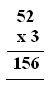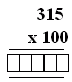Email us to get an instant 20% discount on highly effective K-12 Math & English kwizNET Programs!

#### Online Quiz (WorksheetABCD)

Questions Per Quiz = 2 4 6 8 10

### Grade 3 - Mathematics9.10 Multiplication Review Test

 Q 1: 1453 x 6Answer: Q 2: Expand 3,41230,000+4,000+100+23000+400+10+2300+40+10+2 Q 3: In the multiplication problem shown what are the fators?15652 and 1563 and 15652 and 3 Q 4: Find the product of the multiplication.315315003150 Q 5: 7432 x 6Answer: Q 6: Expand 23,5682,000+300+50+60+820,000+300+500+60+820,000+3,000+500+60+8 Question 7: This question is available to subscribers only! Question 8: This question is available to subscribers only!

#### Subscription to kwizNET Learning System offers the following benefits:

• Unrestricted access to grade appropriate lessons, quizzes, & printable worksheets
• Instant scoring of online quizzes
• Progress tracking and award certificates to keep your student motivated
• Unlimited practice with auto-generated 'WIZ MATH' quizzes